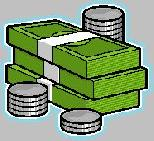Financial Terms Turnover

Information about financial, finance, business, accounting, payroll, inventory, investment, money, inventory control, stock trading, financial advisor, tax advisor, credit.

# Definition of Turnover## Turnover

Mutual Funds: A measure of trading activity during the previous year, expressed as a percentage of
the average total assets of the fund. A turnover ratio of 25% means that the value of trades represented onefourth
of the assets of the fund. Finance: The number of times a given asset, such as inventory, is replaced
during the accounting period, usually a year. Corporate: The ratio of annual sales to net worth, representing
the extent to which a company can growth without outside capital. Markets: The volume of shares traded as a
percent of total shares listed during a specified period, usually a day or a year. Great Britain: total revenue.

## Turnover

The business income or sales of goods and services.

# Related Terms:

## Accounts receivable turnover

The ratio of net credit sales to average accounts receivable, a measure of how
quickly customers pay their bills.

## accounts receivable turnover ratio

A ratio computed by dividing annual
sales revenue by the year-end balance of accounts receivable. Technically
speaking, to calculate this ratio the amount of annual credit sales should
be divided by the average accounts receivable balance, but this information
is not readily available from external financial statements. For
reporting internally to managers, this ratio should be refined and finetuned
to be as accurate as possible.

## Asset turnover

The ratio of net sales to total assets.

## asset turnover

a ratio measuring asset productivity and showing the number of sales dollars generated by each dollar of assets

## asset turnover ratio

A broad-gauge ratio computed by dividing annual
sales revenue by total assets. It is a rough measure of the sales-generating
power of assets. The idea is that assets are used to make sales, and the
sales should lead to profit. The ultimate test is not sales revenue on
assets, but the profit earned on assets as measured by the return on
assets (ROA) ratio.

## Cash Turnover

The number of cash cycles completed in one year.## Fixed asset turnover ratio

The ratio of sales to fixed assets.

## Fixed Assets Turnover Ratio

A measure of the utilization of a company's fixed assets to
generate sales. It is calculated by dividing the sales for the period
by the book value of the net fixed assets.

## Inventory turnover

The ratio of annual sales to average inventory which measures the speed that inventory
is produced and sold. Low turnover is an unhealthy sign, indicating excess stocks and/or poor sales.

## INVENTORY TURNOVER

The number of times a company sold out and replaced its average stock of goods in a year. The formula is:
(Cost of goods sold) / (Average inventory (beginning inventory + ending)/2 )

## Inventory turnover

The number of times per year that an entire inventory or a
subset thereof is used.

## Inventory Turnover

Ratio of annual sales to inventory, which shows how many times the inventory of a firm is sold and replaced during an accounting period.

## inventory turnover ratio

The cost-of-goods-sold expense for a given
period (usually one year) divided by the cost of inventories. The ratio
depends on how long products are held in stock on average before they
are sold. Managers should closely monitor this ratio.

## Inventory Turnover Ratio

Provides a measure of how often a company's inventory is sold or
"turned over" during a period. It is calculated by dividing the sales
figure for the period by the book value of the inventory at the end of
the period.

## Portfolio turnover rate

For an investment company, an annualized rate found by dividing the lesser of
purchases and sales by the average of portfolio assets.## Receivables turnover ratio

Total operating revenues divided by average receivables. Used to measure how
effectively a firm is managing its accounts receivable.

## Total asset turnover

The ratio of net sales to total assets.

## Total Asset Turnover Ratio

A measure of the utilization of all of a company's assets to
generate sales. It is calculated by dividing the sales figure for the
period by the book value of the net fixed assets.

## Du Pont

model a model that indicates the return on investment
as it is affected by profit margin and asset turnover

## Dupont system of financial control

Highlights the fact that return on assets (ROA) can be expressed in terms
of the profit margin and asset turnover.

## Short-term solvency ratios

Ratios used to judge the adequacy of liquid assets for meeting short-term
obligations as they come due, including
1) the current ratio,
2) the acid-test ratio,
3) the inventory turnover ratio, and
4) the accounts receivable turnover ratio.

## Slow-moving item

An inventory item having a slower rate of turnover than the
average turnover for the entire inventory.

## Total revenue

Total sales and other revenue for the period shown. Known as "turnover" in the UK.

Related to : financial, finance, business, accounting, payroll, inventory, investment, money, inventory control, stock trading, financial advisor, tax advisor, credit.

Copyright© 2021 www.finance-lib.com